Next: 6. Convergence and Damping Up: 5. Contacts and Boundaries Previous: 5.8 Mixed-Mode

# 5.9 Thermal Simulation

As thermal circuit simulation is an equivalent problem to electrical circuit simulation MINIMOS-NT makes use of similar formulations. The thermal heat flow over the contact replaces the electrical current and the contact temperature the contact voltage, hence the contact condition reads

 fTC = TC -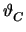= 0 (5.29)

with TC being the contact temperature andbeing the thermal equivalent to the node voltage, the node temperature.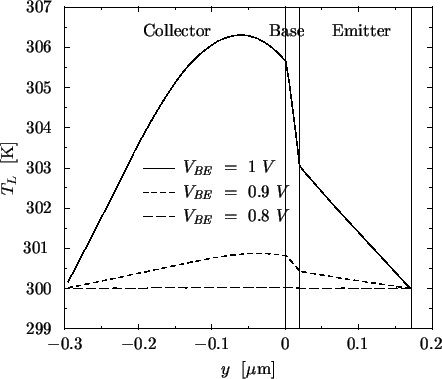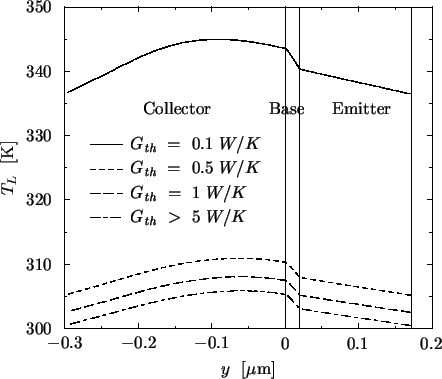In MINIMOS-NT two different thermal contact models are implemented. The first model implements an isothermal contact by simply setting the lattice temperature at the interface points equal to the contact temperature

 TL = TC   . (5.30)

The second model considers a thermal contact resistance and the thermal heat flow density SL at the contact boundary and reads

 SL = n .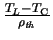(5.31)

with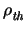being the thermal contact resistivity. The contact conductance Gth is related to the thermal resistivity by Gth = A/with A as the contact area.

The choice of the contact model has a fundamental influence on both the electrical and the thermal behavior of the device. Both models result in approximately the same temperature difference inside the device relative to the boundary values. However, the absolute temperature values can be completely different. Simulated temperature distributions inside the device for the HBT from Section 7 are shown in Fig. 5.4 and Fig. 5.5 for the isothermal and the resistance contact model, respectively, with VCE = 3.5 V. Fig. 5.4 shows the temperature distribution for different base-emitter voltages VBE whereas for Fig. 5.5 VBE = 1.0 V was used and Gth was varied. For VBE = 1.0 V both contact models generate the same relative temperature distribution but in the case of the resistance contact model the temperature is shifted by an offset which exponentially depends on Gth. For Gth as small as 10 mW/K no solution could be found at all as the lattice temperature would exceed 600 K which inhibits a successful simulation. Although VBE = 1.0 V is quite high it must be pointed out that even for lower bias conditions the same situation occurs for improper choice of Gth.

These investigations show that the isothermal model can only be used when the exact contact temperatures are known. Simply assuming ambient temperature delivers completely wrong results as the simulated region of the device is normally reduced to the electrically active region which is only a small portion of the whole geometry. Hence, especially for mixed-mode simulations use of thermal contact resistances is mandatory.

In Fig. 5.6 the heat generation inside the device is shown for different values of Gth. As the current density remains approximately constant within this cross-section, the maximum of the heat generation is located at the base-collector space charge region where the electric field is maximal. As VCE = 3.5 V was assumed which is quite moderate, even higher heat generation rates can be expected for the circuits simulated in Section 7. Although the final values may give reasonable temperature distributions, during iteration the bias voltages of the device may vary considerably and can easily exceed VBE = 1.5 V and VCE = 20 V. This situation can occur during mixed-mode simulation of circuits with large supply voltages. Under these bias conditions, the thermal problem cannot be solved as the melting point of the device would be exceeded considerably. This causes excessive problems when simulating fully-coupled electro-thermal systems especially because measured values for Gth are in the range 1-10 mW/K.

In Fig. 5.7 the temperature distribution for different base-emitter voltages is shown generated with a quite large value of 100 mW/K for Gth. All these figures indicate, that the temperature difference inside the device is normally much smaller than the temperature difference induced by the contact model. For some simulations it might therefore be beneficial to substitute the heat flow equation by a spatially constant lattice temperature which is determined by the dissipated power of the device and thermal resistances at the contacts. For example, when the device is known to operate at 400 K, the local generated heat may be neglected.

Figure 5.6: Heat generation distribution of a HBT for different thermal contact conductances.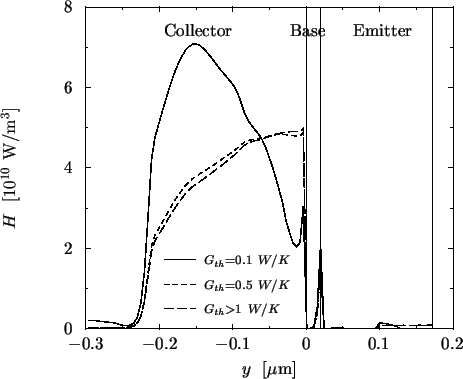Next: 6. Convergence and Damping Up: 5. Contacts and Boundaries Previous: 5.8 Mixed-Mode
Tibor Grasser
1999-05-31